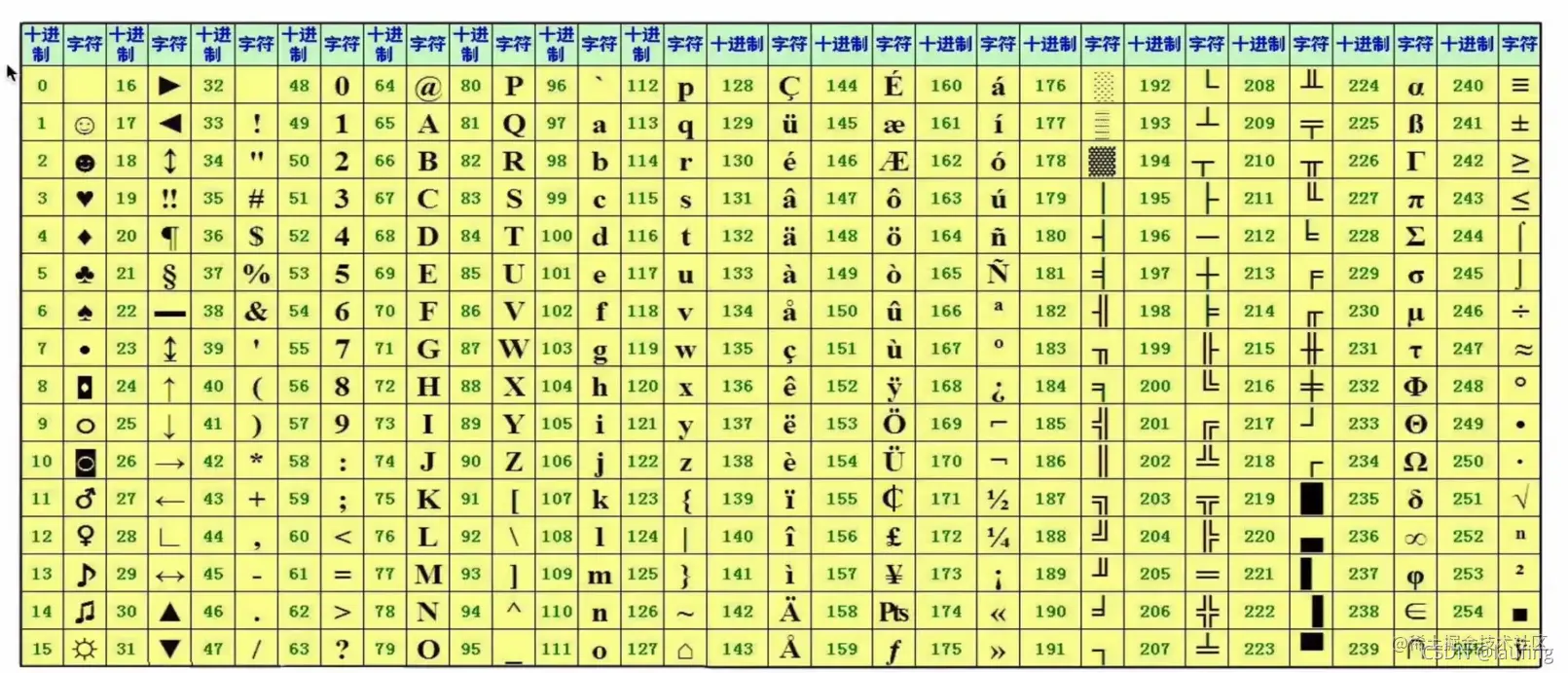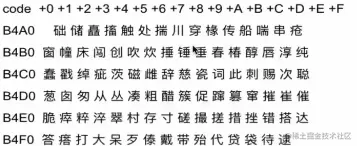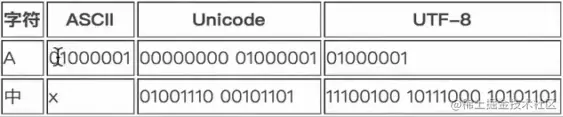# Python and binary

2022-01-31 17:22:16 laufing

# Decimal and binary

Decimal system ---> Binary system , except 2 The remainder is inverted
Like the decimal system 5, Represented as binary 101 python Realization ：

``````d = 5
bin(5)
#"0b101"

# Convert the current binary data , To integer
int("0b101",base=2) # 5
Copy code ``````

The fractional part , Then take 2 Round and align
Like the decimal system 0.5, Represented as binary 0.1

Binary system ----> Decimal system , Just weighted sum
Such as binary 101, Its decimal system is expressed as 1*2^2^+0*2^1^+1*2^0^=5

# Decimal and octal

Decimal system ----> octal , except 8 The remainder is inverted
Like the decimal system 8 , Expressed in octal 0o10

``````d = 8
oct(d)
#0o10
int("0o10",base=8)
Copy code ``````

octal ---> Decimal system , Similarly, weighted summation

Decimal system ----> Hexadecimal ,same

``````d = 17
hex(d)
#'0x11'
int("0x11",base=16)  # Hexadecimal to decimal , To sum by weight
#1*16^1 + 1*16^0
Copy code ``````

# Binary representation of characters

The character has a corresponding ASCII clock , The corresponding decimal number , Change to binary``````# Get the binary of English characters
bin(ord("a"))
#'0b1100001', One byte
chr
Copy code ``````

1byte = 8bit 1Kb = 1024bytes 1Mb = 1024Kb ...

# Chinese and binary

Chinese characters have corresponding GBK/GB2312/GB18030 Encoding table , Corresponding decimal system , Change to binary .A Chinese character takes up two bytes , Now the mainstream is GBK code .

Universal coding method Unicode, Correspond all characters to binary , But each character takes == Two bytes == Of memory , Than ASCII Double the code , Temporarily stored in memory can also , Saving to disk or network transmission is a waste of space .

So there is a utf-8 The coding ,Unicode Transform Format, Save disk 、 Space during transmission .Data in memory -->Unicode code ----2bytes/per char
Data on disk -->UTF-8----1byte for english, 3bytes for chinese

linux Type... On the command line locale, View supported encoding formats

# py2 And py3 The coding

py2 In memory is ASCII code , If you use Chinese, you need to declare

linux/macOS The system uses utf-8

``````#！/usr/bin/python2
# -*- coding:utf-8 -*-
print(" chinese ")
Copy code ``````

windows System use gbk code

py3 In memory is Unicode code

# hash Hash

Put different inputs , Hash calculation yields a fixed length output .

characteristic ： Irreversible Fast calculation , Even if the file is big , It won't affect the calculation speed too much The type of data entered , Must be immutable

purpose ： Password encryption File check

``````hash("xxxxx")

from hashlib import md5
m = md5()
m.update("xxx".encode())
m.hexdigest()
Copy code ``````

# python Based on hash Data type of

dict/set Why is dictionary query fast ？ In the dictionary , Every key Have to go through hash Calculation , For all the key Of hash Value to sort . Looking for some key The value of , First calculate its hash value , According to its hash Values are in the already ordered hash Binary search in value sequence . fast

According to the query hash value , Get the corresponding value The index of .

Why? set Automatic weight removal ？ When saving value , Calculating the hash value , Then store the data in the corresponding position , If there is no data at this location , The insert ; Otherwise do not insert .

Last one ：python Data types continued